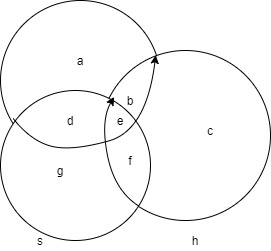Filters
Latest Questions
Mathematics
Properties of sets
Using properties of sets, show that
(i) $A \cup (A \cap B) = A$
(ii) $A \cap (A \cup B) = A$

Mathematics
Properties of sets
State whether the following pairs of sets are equal or not:
$A=\left\{ 2,4,6,8,10 \right\}$ , $B=\left\{ \text{even natural numbers} \right\}$

Mathematics
Properties of sets
In a class of 45 pupils, 21 play chess, 23 play cards and 5 play both the games. Find
(i)How many do not play any of the games;
(ii)How many play chess only;

Mathematics
Properties of sets
Let A and B be two sets such that $n\left( A \right)=3$ and $n\left( B \right)=2$. If $\left( x,1 \right)$ , $\left( y,2 \right)$ , $\left( z,1 \right)$ are in $A\times B$ , write A and B?
Mathematics
Properties of sets
Let $A$ and $B$ be two sets such that $n\left( A \right) = 3$ and$n\left( B \right) = 2$. If $\left( {x,1} \right),\left( {y,2} \right),\left( {z,1} \right)$ are in $A \times B$, write $A$ and $B$.
Mathematics
Properties of sets
Find the correct answer $A\Delta B=$ 
A. $\left( A\bigcup B \right)-\left( A\bigcap B \right)$ 
B. $\left( A\bigcup B \right)+\left( A\bigcap B \right)$ 
C. $\left( A\bigcap B \right)-\left( A\bigcup B \right)$ 
D. None of these 
Mathematics
Properties of sets
Out of 500 first year students, 260 passed in the first semester and 210 passed in the second semester. If 170 did not pass in either semester, how many passed in both semester?
A. 30
B. 40
C. 70
D. 140
Mathematics
Properties of sets
Let X be a family of sets and R be a relation on X defined as ‘A is disjoint from B’. Then R is
(a) Reflexive
(b) Symmetric
(c) Anti – symmetric
(d) Transitive.
Mathematics
Properties of sets
The number of subsets of $\left\{ {1,2,...,9} \right\}$ containing at least one odd number is
a.324
b.396
c.496
d.512

Mathematics
Properties of sets
Out of 100 students, 15 passed in English, 12 passed in Mathematics, 8 in Science, 6 in English & Mathematics, 7Mathematics and Science, 4 English & Science, 4 in all the three. Find how many passes1.In English & Mathematics but not in Science
2.In Mathematics & Science but not in English
3.In only Mathematics
4.In more than one subject only

Mathematics
Properties of sets
Which of the following collections are sets?
(i) the collection of prime numbers
(ii) the collection of easy sub topics in this chapter
(iii) the collection of good teachers in your school.
(iv) the collection of girls in your class.
(v) the collection of odd natural numbers

A. (i), (ii), (iv) only.
B. (i), (iv), (v) only.
C. (ii), (iii), (v) only.
D. (iii), (iv) only.
Mathematics
Properties of sets
Examine whether the following statements are true or false:
(i) $\left\{ {a,b} \right\} \not\subset \left\{ {b,c,a} \right\}$
(ii) $\left\{ {a,e} \right\} \subset \{ x:{\text{ }}x{\text{ }}is{\text{ }}a{\text{ }}vowel{\text{ }}in{\text{ }}the{\text{ }}English{\text{ }}alphabet)$
(iii) $\left\{ {1,2,3} \right\} \subset \left\{ {1,3,5} \right\}$
(iv) $\left\{ a \right\} \subset \left\{ {a,b,c} \right\}$
(v) $\left\{ a \right\} \in \left\{ {a,b,c} \right\}$
(vi) $\left\{ {x:x{\text{ }}is{\text{ }}an{\text{ }}even{\text{ }}natural{\text{ }}number{\text{ }}less{\text{ }}than{\text{ }}6} \right\}$ $\subset \left\{ {x:x{\text{ }}is{\text{ }}a{\text{ }}natural{\text{ }}number{\text{ }}which{\text{ }}divides{\text{ }}36} \right\}$

Prev
1
2